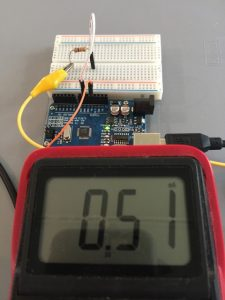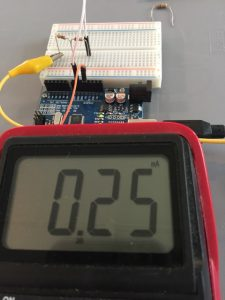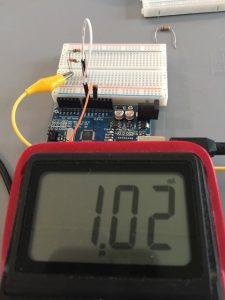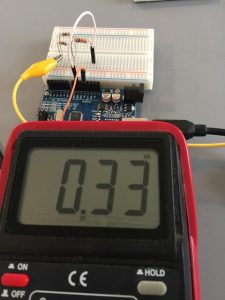# Calculating Current

## Introduction to Calculating Current (Amps)

In the last post, we discussed how to calculate resistance.   If you are unfamiliar with calculating resistance, please review the post before continuing.  In this post, we will learn to calculate current. We talked about a variable resistance being similar to the water faucet in your house.   Current would relate to the amount of flow (gallons per minute) coming out of your faucet.    If we know the Voltage (similar to pressure), and the resistance, we can calculate the amount of current in Amperes.

It’s important to know the current so we can size our wiring, fuses, and other components correctly in our circuit.   The Atmega 328 (as on the Uno board) can handle up to 40mA (MilliAmps) on an output.   If our circuit draws more than 40mA from the Atmega 328 we will damage the microprocessor.

## Ohm’s Law

Current is expressed by the variable I in Ohm’s Law.   I=V/R.   Consider our 10K (Kilo Ohm) resistor.   If we apply 5V across the 10K resistor, Our current should be 5/10,000 or .0005 Amps (.5 MiliAmps).   Let’s check the meter to see if this is the case.When we had the resistors in series, we had a total of 20K.  5v/20K would be .25mA:Now, remember when we connected the two 10K resistors in parallel, our total resistance was 5K.  When we do our calculations, we will take 5v/5K, and we will have .1mA flowing through our resistor bank.   There is still .5mA flowing through each resistor because they are both supplied with 5V, so the total current (It) is .1mA.   Let’s check this on the meter.Next, we have a series/parallel combination.   We have two 10K resistors in parallel, then the parallel branch is in series with another 10K resistor for a total of 15K.    If we take 5v/15k, we will have .33mA.   Again, there is nothing like checking this in the real world, so let’s look at the meter for this circuit:Our Ammeter is connected in series in this case, so when using a multimeter, you will need to take the proper precautions.   The ammeter has a very low resistance, acting as a short, so never connect it directly across a power supply.   Also, be sure to use a meter that is rated for the amperage of your circuit, and that the ammeter is connected properly.

If you would like on-site industrial training, please visit my employer’s website!

— Ricky Bryce